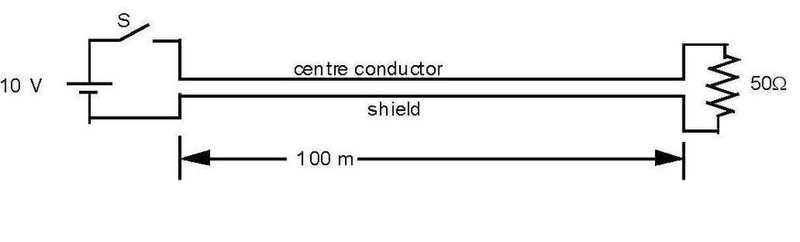# Coaxial cable; current problem

mathman44

## Homework Statement

Type P241B coaxial cable has an inductance of 0.4 μH/m and a capacitance of 40 pF/m.
The diagram below shows a 100 m length of this cable connected to a battery and switch at the sending end and a 50Ω resistor at the receiving end.
(a) If S is closed, what initial current will flow from the battery?
(b) How long will it take before this current changes?
(c) What will be the battery current at t=∞?## The Attempt at a Solution

I calculated the effective resistance of the cable to be 100 ohms. Since this is in series with a 50 ohm resistor then the total resistance is 150.

Last edited:

mathman44
does this sound reasonable?

a) at t=0 current is zero because of inductor
b)
c) at t=infinity current is zero again because of capacitor

I'm stuck on B :s

Mindscrape
Hah, yeah, you got the easy parts right :p

The other part is simply KVL: add up all the potentials going along the circuit to get your differential equation, and then solve the ODE. Depending on how complex your class is, you may need to solve simultaneous ODEs.

mathman44
Thanks for the response! I don't think I know what you're talking about for the second part, though... at any rate, it doesn't relate to our class material. Might there be another way to solve this?